Question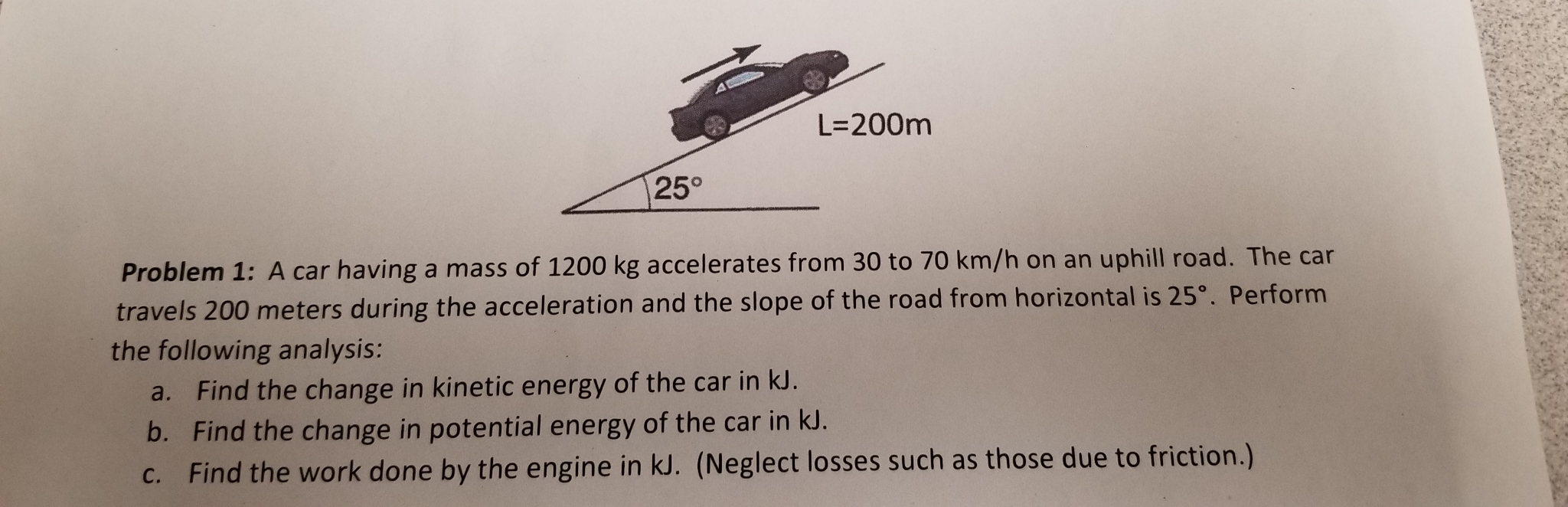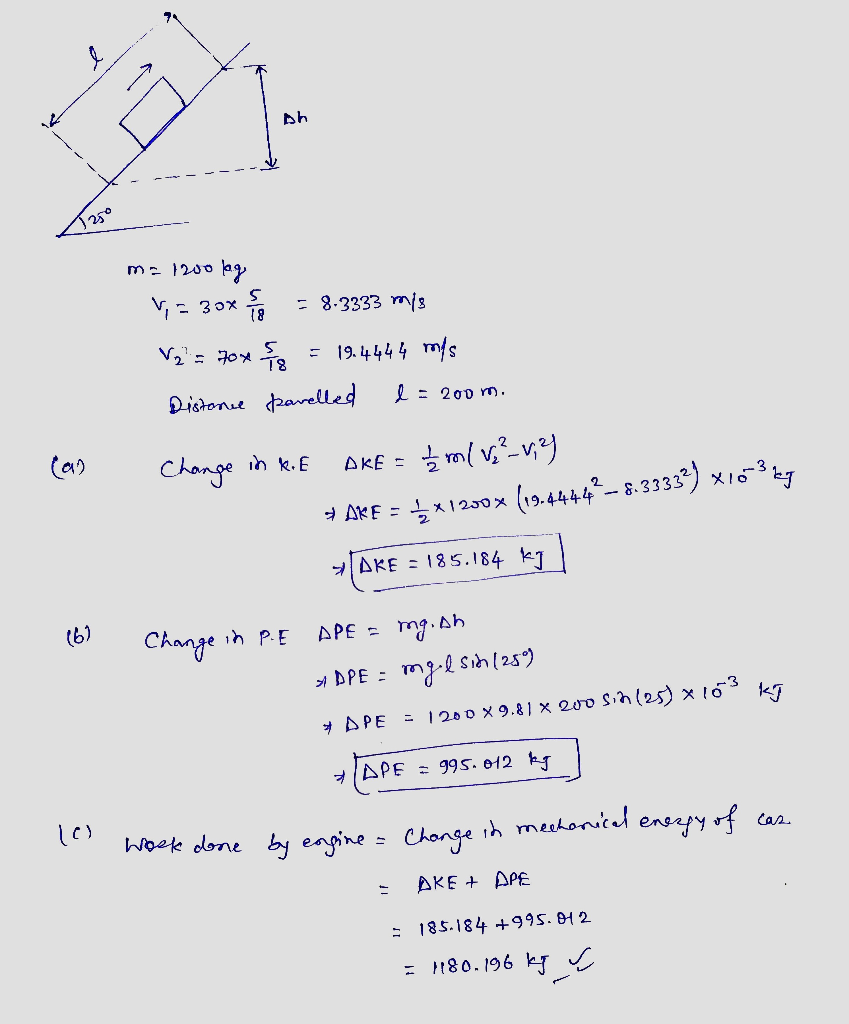#### Earn Coins

Coins can be redeemed for fabulous gifts.

Similar Homework Help Questions
• ### An automobile has a mass of 1200 kg. What is its kinetic energy, in kJ, relative...

An automobile has a mass of 1200 kg. What is its kinetic energy, in kJ, relative to the road when traveling at a velocity of 50 km/h? If the vehicle accelerates to 100 km/h in 15 seconds, what is the change in power required?

• ### A 1250 kg car drives up a hill that is 16.2 m high. During the drive,...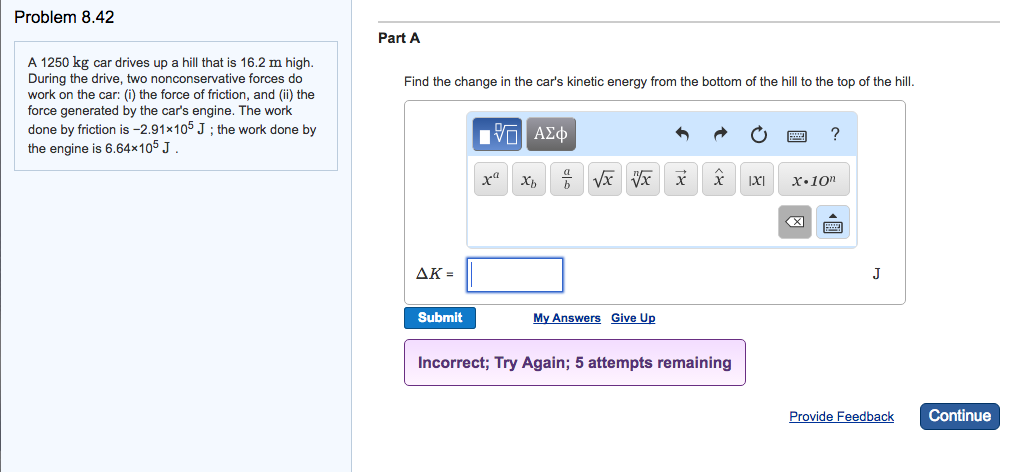A 1250 kg car drives up a hill that is 16.2 m high. During the drive, two nonconservative forces do work on the car: (i) the force of friction, and (ii) the force generated by the car's engine. The work done by friction is -2.91 times 10^5 J; the work done by the engine is 6.64 times 10^5 J. Find the change in the car's kinetic energy from the bottom of the hill to the top of the hill.

• ### 3. An engine exerts a force of 10000 N on a 1000 kg car travelling over...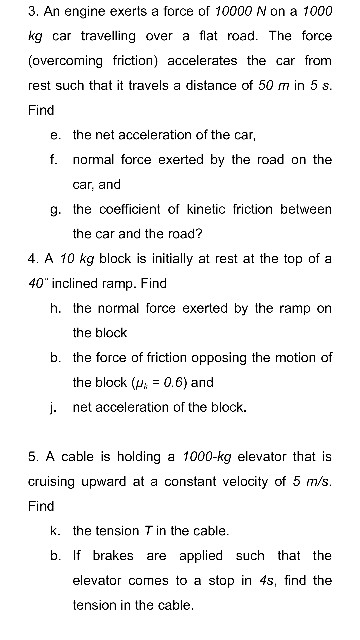3. An engine exerts a force of 10000 N on a 1000 kg car travelling over a flat road. The force (overcoming friction) accelerates the car from rest such that it travels a distance of 50 m in 5 s Find e. the net acceleration of the car, f. normal force exerted by the road on the car, and g. the coefficient of kinetic friction between the car and the road? 4. A 10 kg block is initially at rest...

• ### A 1500-kg car starts from rest and accelerates uniformly to 18.0 m/s in 12.0 s over...

A 1500-kg car starts from rest and accelerates uniformly to 18.0 m/s in 12.0 s over a dry concrete road. Assume that kinetic friction remains constant at 400 N during this time. – (a) Find the net work done on the car and the work done by the engine. – (b) How many revolutions does each tire make over the 12.0 s interval? What is the angular speed of the wheels when the car has traveled half the total distance?...

• ### A 0 4 kg flywheel, I = 1/2mr^2, accelerates from rest to 1200 rev/min in 6.0...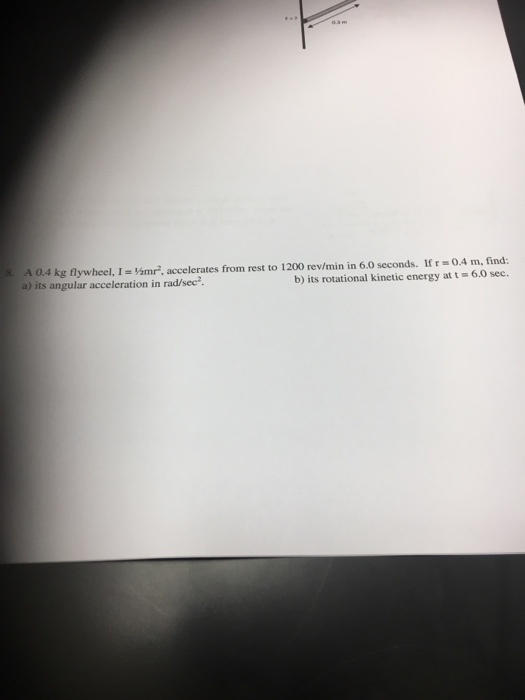A 0 4 kg flywheel, I = 1/2mr^2, accelerates from rest to 1200 rev/min in 6.0 seconds. If r = 0.4 m, find: a) its angular acceleration in rad/sec^2 b) its rotational kinetic energy at t = 6.0 sec.

• ### A red car with a 70kW engine and a mass of 1335kg accelerates at full power for 2s from rest along a level stretch of road heading east before going down a 3m high hill

A red car with a 70kW engine and a mass of 1335kg accelerates at full power for 2s from rest along a level stretch of road heading east before going down a 3m high hill. The driver puts the car in neutral to accelerate as they coast down the hill. Some distance beyond the hill on another level stretch of road is an intersection. A blue car of mass 1800kg drives south at 60km/h into this intersection in front of...

• ### 1. A car accelerates from zero to 105 km/h in 5.21 s. What is its average...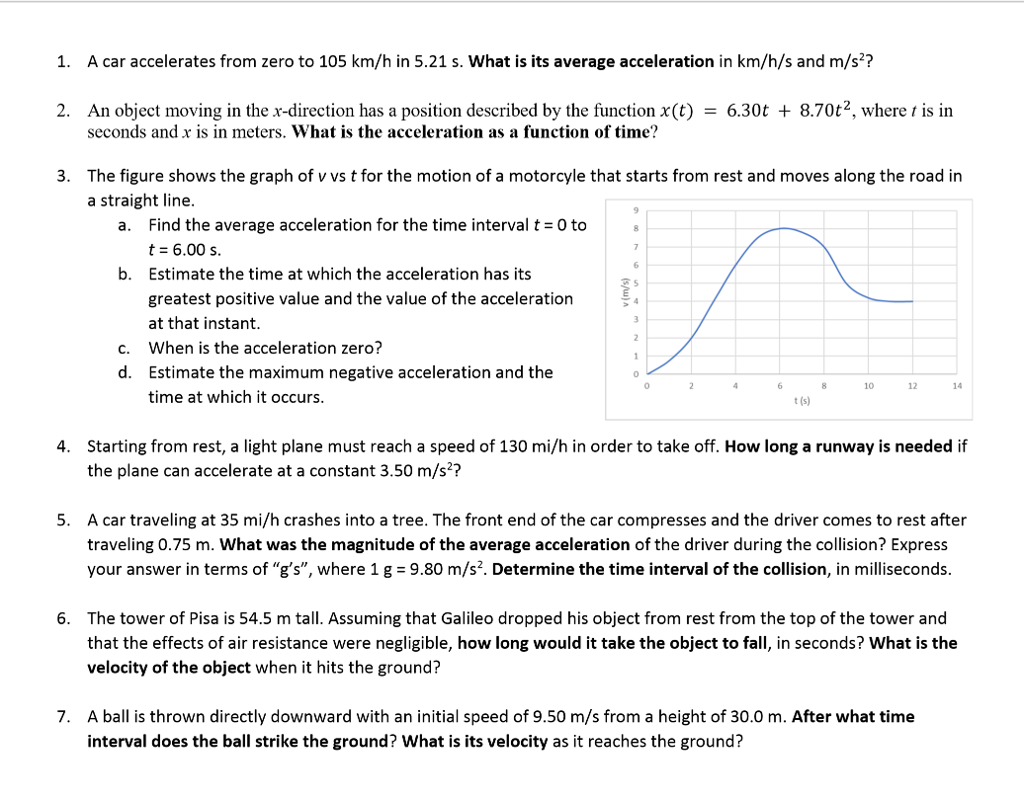1. A car accelerates from zero to 105 km/h in 5.21 s. What is its average acceleration in km/h/s and m/s? 2. An object moving in the x-direction has a position described by the function x(t) - 6.30t + 8.70t2, where t is in seconds and x is in meters. What is the acceleration as a function of time? The figure shows the graph of v vs t for the motion of a motorcyle that starts from rest and moves...

• ### help with part 5 1. A 125-kg load is lifted 12.0 m vertically with an acceleration...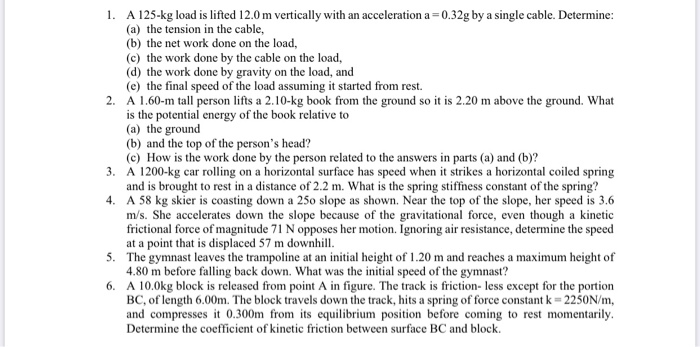help with part 5 1. A 125-kg load is lifted 12.0 m vertically with an acceleration a -0.32g by a single cable. Determine: (a) the tension in the cable, (b) the net work done on the load, (c) the work done by the cable on the load, (d) the work done by gravity on the load, and (e) the final speed of the load assuming it started from rest. 2. A 1.60-m tall person lifts a 2.10-kg book from the...

• ### 1. A pole is held vertically by attaching wires at a height of 13.4 m above...

1. A pole is held vertically by attaching wires at a height of 13.4 m above the ground. The other end of each wire is anchored in the ground at a distance of 9.54 m from the base of the pole. The pole makes a right angle with the ground. What is the length of each wire? 2. A bug crawls 2.25 m along the base of a wall. Upon reaching a corner, the bug's direction of travel changes from...